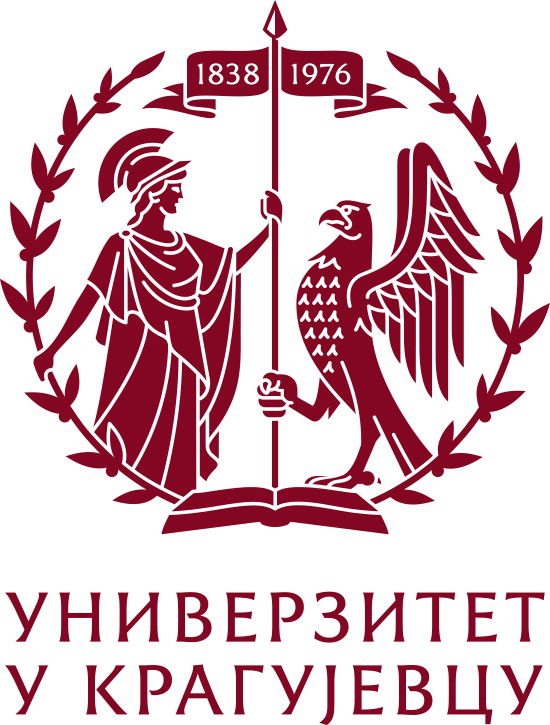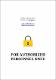Please use this identifier to cite or link to this item: `https://scidar.kg.ac.rs/handle/123456789/9866`
 Title: On the Zagreb indices equality Authors: Abdo H.Dimitrov D.Gutman, IvanIssue Date: 2012 Abstract: For a simple graph G=(V,E) with n vertices and m edges, the first Zagreb index and the second Zagreb index are defined as M1(G)= ∑v∈Vd(v)2 and M2(G)= ∑uv∈Ed(u)d(v), where d(u) is the degree of a vertex u of G. In , it was shown that if a connected graph G has maximal degree 4, then G satisfies M1(G)n=M2(G)m (also known as the Zagreb indices equality) if and only if G is regular or biregular of class 1 (a biregular graph whose no two vertices of same degree are adjacent). There, it was also shown that there exist infinitely many connected graphs of maximal degree Δ=5 that are neither regular nor biregular of class 1 which satisfy the Zagreb indices equality. Here, we generalize that result by showing that there exist infinitely many connected graphs of maximal degree Δ<5 that are neither regular nor biregular graphs of class 1 which satisfy the Zagreb indices equality. We also consider when the above equality holds when the degrees of vertices of a given graph are in a prescribed interval of integers. © 2011 Elsevier B.V. All rights reserved. URI: https://scidar.kg.ac.rs/handle/123456789/9866 Type: article DOI: 10.1016/j.dam.2011.10.003 ISSN: 0166-218X SCOPUS: 2-s2.0-82755184117 Appears in Collections: Faculty of Science, Kragujevac#### Page views(s)

105

29.86 kBAdobe PDF